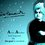# INPHO -2005

Rolling sphere :-

A sphere of radius R is projected up an inclined plane of angle $\theta$ with initial speed u and angular velocity ${ \omega }_{ 0 }$ in the direction such that it will roll up. The coefficient of friction is $tan(\theta )/7$ and it is given that u> ${ \omega }_{ 0 }$ . Give the direction of friction for different parts of motion as sphere rises. Obtain the time for which sphere moves up the plane till it stops.Note by Tushar Gopalka
6 years, 6 months ago

This discussion board is a place to discuss our Daily Challenges and the math and science related to those challenges. Explanations are more than just a solution — they should explain the steps and thinking strategies that you used to obtain the solution. Comments should further the discussion of math and science.

When posting on Brilliant:

• Use the emojis to react to an explanation, whether you're congratulating a job well done , or just really confused .
• Ask specific questions about the challenge or the steps in somebody's explanation. Well-posed questions can add a lot to the discussion, but posting "I don't understand!" doesn't help anyone.
• Try to contribute something new to the discussion, whether it is an extension, generalization or other idea related to the challenge.

MarkdownAppears as
*italics* or _italics_ italics
**bold** or __bold__ bold
- bulleted- list
• bulleted
• list
1. numbered2. list
1. numbered
2. list
Note: you must add a full line of space before and after lists for them to show up correctly
paragraph 1paragraph 2

paragraph 1

paragraph 2

[example link](https://brilliant.org)example link
> This is a quote
This is a quote
    # I indented these lines
# 4 spaces, and now they show
# up as a code block.

print "hello world"
# I indented these lines
# 4 spaces, and now they show
# up as a code block.

print "hello world"
MathAppears as
Remember to wrap math in $$ ... $$ or $ ... $ to ensure proper formatting.
2 \times 3 $2 \times 3$
2^{34} $2^{34}$
a_{i-1} $a_{i-1}$
\frac{2}{3} $\frac{2}{3}$
\sqrt{2} $\sqrt{2}$
\sum_{i=1}^3 $\sum_{i=1}^3$
\sin \theta $\sin \theta$
\boxed{123} $\boxed{123}$

Sort by:

- 6 years, 6 months ago

Is the time $\frac { 19u+2R{ \omega }_{ 0 } }{ 21gsin{ \theta } }$ ????

- 6 years, 6 months ago

How did you solve it?

- 6 years, 6 months ago

- 6 years, 6 months ago

\bump

- 1 year, 8 months ago

@Mvs Saketh , @Ronak Agarwal @Deepanshu Gupta @jatin yadav @Calvin Lin @David Mattingly , its an awesome question. Solve it and enjoy.

- 6 years, 6 months ago